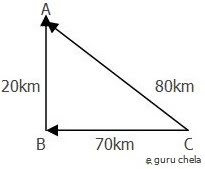# Average velocity and average speed

A distance and displacement have distinctly different meanings so do speed and velocity. A fast-moving object has a high speed and covers a relatively large distance in a short amount of time. Contrast this to a slow-moving object that has a low speed; it covers a relatively small amount of distance in the same amount of time. An object with no movement at all has a zero speed. Speed is a scalar quantity that refers to how fast an object is moving. Speed can be thought of as the rate at which an object covers distance.If first 70 km to B and then 20 km to A .if u drove an average speed of 40 km per hr and you have to cover total 70+20=90 km. trip will take 1.30 hr so we will calculate

80 km / 1.30hr = 60 km/ hr

### Calculating Average Speed and Average Velocity

If we will calculate the Average speed and average Velocity then we should to know total distance of traveling way and what did take time of travel

So ,let's see the formulae of it :
Average speed = total distance traveled /total time of travel

#### The average velocity is often describe using this formula

Average Velocity = position of A / time = displacement / time

### Example :

A is driving bike and distance of goal is 200 km .His trip take 5 hr then what will be the average speed to A ?

we know that v =d / t

=200km / 5 hr

=40 km/hr

### Similarities Between Average Speed and Average Velocity

Both terms are average of some length by time taken together. Formula for calculating average speed and average velocity is almost samilar .The SI unit for measurement of both are same.

### Difference between average velocity and average speed

1. Average speed is a scalar quantity on the other hand average velocity is a vector quantity.

2. Average speed concerned total distance traveled while average velocity is concerned with the displacement between two points.

### Average Velocity defination

The average velocity of an object is its total displacement divided by the total time taken. In simple words, it is the rate at which an object changes its position from one place to another. Average velocity is a Vector quantity. The SI unit is meters per second. However, any distance unit per any time unit can be used when necessary, such as miles per hour (mph) or kilometer per hour (kmph).

### Average Speed defination

Average speed equals total distance covered divided by total time to cover the given distance.Speed is a scalar quantity and it has no direction. High speed meaning is that particle is moving fast and if it is low meaning is that particle is moving slow.